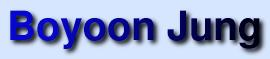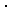Boyoon Jung's Library Manual

# bj::VFH Class Reference [Robot Programming]

A Vector Field Histogram (VFH) algorithm. More...

`#include <vfh.h>`

Collaboration diagram for bj::VFH:[legend]
List of all members.

## Public Member Functions

VFH (double radius=3.0, double resolution=5 *M_PI/180, double robot_radius=0.5, double min_distance=0.1, double threshold_high=17.0, double threshold_low=12.0, int threshold_wide=16)
A constructor.
~VFH (void)
A destructor.
void update (double distance, double direction, double ox=0.0, double oy=0.0, double oa=0.0, int increase=50, int decrease=25)
Update the local occupancy grid map.
void set (double rx, double ry, double ra)
Set a new robot pose.
void get (double tx, double ty, double &linear, double &angular)
void getPolar (double td, double ta, double &linear, double &angular)
void setParams (double radius=3.0, double resolution=5 *M_PI/180, double robot_radius=0.5, double min_distance=0.1, double threshold_high=17.0, double threshold_low=12.0, int threshold_wide=16)
Set the parameters for VFH algorithm.
void setThresholdParams (double threshold_high, double threshold_low, int threshold_wide=16)
Set the threshold parameters.
void setVelocityParams (double max_linear=0.3, double max_angular=90 *M_PI/180, double min_linear=0.05, double min_angular=5 *M_PI/180)
Set the limit of velocities.
void setCostParams (double goal=5, double direction=2, double previous=2)
Set the cost function parameters.
void setMapParams (double map_size=5.0, double grid_size=0.1, double move_step=1.0)
Set the parameters for a local occupancy grid map.
double x (void)
Return the current robot position on X axis.
double y (void)
Return the current robot position on Y axis.
OccupancyMapmap (void)
Return the current occupancy map.
double mapGridSize (void)
Return the grid size of the occupancy map.
double * histogram (void)
Return the polar histogram.
bool * binHistogram (void)
Return the binary polar histogram.
int histGridSize (void)
Return the grid size of the polar histograms.
bool writeTargetPosition (int file, double tx, double ty)
Write the target position into a file (for debugging).
bool writeMap (int file)
Write the occupancy grid map data into a file (for debugging).
bool writeHistogram (int file)
Write the polar histogram into a file (for debugging).

## Protected Member Functions

void update_vector_magnitude (void)
Update the vector magnitude of an active cells.
void update_polar_histogram (void)
Update the polar histogram.
void select_candidate_directions (double tx, double ty)
Select candidate directions based on the polar histogram.
double select_best_direction (void)
Select the best direction based on the cost function.
void initialize_active_region (void)
Initialize an active region (pre-computation for fast execution).
int angle2idx (double a)
Convert an angle into a histogram index.
int idx2pidx (int i)
Convert a histogram index into an angle.
double cost_function (double a, double t, double c, double p)
Cost function.
double R2_2PI (double r)
Convert to a radian in 0 ~ 2*pi.
double R2_PI (double r)
Convert to a radian in -pi ~ pi.

## Detailed Description

A Vector Field Histogram (VFH) algorithm.

VFH class implements VFH+ algorithm. There is one modification from the original algorithm; (r^2 / d^2) is used instead of (a - b*d^2).

There are three steps:

• invoke set() when the position of a robot has been updated.
• invoke update() when distance measurements are available.
• invoke get() to retrieve recommanded motor commands.

## Constructor & Destructor Documentation

 bj::VFH::VFH ( double radius = `3.0`, double resolution = `5 *M_PI/180`, double robot_radius = `0.5`, double min_distance = `0.1`, double threshold_high = `17.0`, double threshold_low = `12.0`, int threshold_wide = `16` )

A constructor.

Parameters:
 radius The radius of an active region. [in meters] resolution The angular resolution of polar histograms. [in radians] robot_radius The radius of a robot body. [in meters] min_distance The minimum distance between a robot and objects. [in meters] threshold_high The upper threshold for binary polar histograms. threshold_low The lower threshold for binary polar histograms. threshold_wide The threshold for wide/narrow opening.

 bj::VFH::~VFH ( void )
 A destructor.

## Member Function Documentation

 int bj::VFH::angle2idx ( double a ) ` [inline, protected]`
 Convert an angle into a histogram index.

 bool* bj::VFH::binHistogram ( void ) ` [inline]`
 Return the binary polar histogram.

 double bj::VFH::cost_function ( double a, double t, double c, double p ) ` [inline, protected]`
 Cost function.

 void bj::VFH::get ( double tx, double ty, double & linear, double & angular ) ` [inline]`

Parameters:
 tx The target position along X axis in the local coordinates. ty The target position along Y axis in the local coordinates. [out] linear The recommended linear velocity. [in meters per second] [out] angular The recommended angular velocity. [in radians per second]

 void bj::VFH::getPolar ( double td, double ta, double & linear, double & angular )

Parameters:
 td The distance to the target position. ta The angle to the target position. [out] linear The recommended linear velocity. [in meters per second] [out] angular The recommended angular velocity. [in radians per second]

 double bj::VFH::heading ( void ) ` [inline]`

 int bj::VFH::histGridSize ( void ) ` [inline]`
 Return the grid size of the polar histograms.

 double* bj::VFH::histogram ( void ) ` [inline]`
 Return the polar histogram.

 int bj::VFH::idx2pidx ( int i ) ` [inline, protected]`
 Convert a histogram index into an angle.

 void bj::VFH::initialize_active_region ( void ) ` [protected]`
 Initialize an active region (pre-computation for fast execution).

 OccupancyMap* bj::VFH::map ( void ) ` [inline]`
 Return the current occupancy map.

 double bj::VFH::mapGridSize ( void ) ` [inline]`
 Return the grid size of the occupancy map.

 double bj::VFH::R2_2PI ( double r ) ` [inline, protected]`
 Convert to a radian in 0 ~ 2*pi.

 double bj::VFH::R2_PI ( double r ) ` [inline, protected]`
 Convert to a radian in -pi ~ pi.

 double bj::VFH::select_best_direction ( void ) ` [protected]`
 Select the best direction based on the cost function.

 void bj::VFH::select_candidate_directions ( double tx, double ty ) ` [protected]`
 Select candidate directions based on the polar histogram.

 void bj::VFH::set ( double rx, double ry, double ra ) ` [inline]`
 Set a new robot pose.

 void bj::VFH::setCostParams ( double goal = `5`, double direction = `2`, double previous = `2` ) ` [inline]`

Set the cost function parameters.

Parameters:
 goal The higher, the more willing to move toward a goal position. direction The higher, the more willing to move in the current direction. previous The higher, the more willing to move in the previous direction.

 void bj::VFH::setMapParams ( double map_size = `5.0`, double grid_size = `0.1`, double move_step = `1.0` )

Set the parameters for a local occupancy grid map.

Parameters:
 map_size The size of a local grid map. [in meters] grid_size The grid size of a map. [in meters] move_step The minimum distance to shift map. [in meters]

 void bj::VFH::setParams ( double radius = `3.0`, double resolution = `5 *M_PI/180`, double robot_radius = `0.5`, double min_distance = `0.1`, double threshold_high = `17.0`, double threshold_low = `12.0`, int threshold_wide = `16` )

Set the parameters for VFH algorithm.

Parameters:
 radius The radius of an active region. [in meters] resolution The angular resolution of polar histograms. [in radians] robot_radius The radius of a robot body. [in meters] min_distance The minimum distance between a robot and objects. [in meters] threshold_high The upper threshold for binary polar histograms. threshold_low The lower threshold for binary polar histograms. threshold_wide The threshold for wide/narrow opening.

 void bj::VFH::setThresholdParams ( double threshold_high, double threshold_low, int threshold_wide = `16` ) ` [inline]`

Set the threshold parameters.

Parameters:
 threshold_high The upper threshold for binary polar histograms. threshold_low The lower threshold for binary polar histograms. threshold_wide The threshold for wide/narrow opening.

 void bj::VFH::setVelocityParams ( double max_linear = `0.3`, double max_angular = `90*M_PI/180`, double min_linear = `0.05`, double min_angular = `5*M_PI/180` ) ` [inline]`

Set the limit of velocities.

Parameters:
 max_linear The maximum linear velocity. [in meters] max_angular The maximum angular velocity. [in radians] min_linear The minimum linear velocity. [in meters] min_angular The minimum angular velocity. [in radians]

 void bj::VFH::update ( double distance, double direction, double ox = `0.0`, double oy = `0.0`, double oa = `0.0`, int increase = `50`, int decrease = `25` ) ` [inline]`

Update the local occupancy grid map.

Parameters:
 distance The distance to the closest object. [in meters] direction The direction of the measurement. [in radians] ox The offset of a sensor along X axis in robot's coordinates. [in meters] oy The offset of a sensor along Y axis in robot's coordinates. [in meters] oa The angular offset of a sensor in robot's coordinates. [in radians] increase An increase for "occupied cell" update. decrease A decrease for "un-occupied cell" update.

 void bj::VFH::update_polar_histogram ( void ) ` [protected]`
 Update the polar histogram.

 void bj::VFH::update_vector_magnitude ( void ) ` [protected]`
 Update the vector magnitude of an active cells.

 bool bj::VFH::writeHistogram ( int file )
 Write the polar histogram into a file (for debugging).

 bool bj::VFH::writeMap ( int file ) ` [inline]`
 Write the occupancy grid map data into a file (for debugging).

 bool bj::VFH::writeTargetPosition ( int file, double tx, double ty )
 Write the target position into a file (for debugging).

 double bj::VFH::x ( void ) ` [inline]`
 Return the current robot position on X axis.

 double bj::VFH::y ( void ) ` [inline]`
 Return the current robot position on Y axis.

The documentation for this class was generated from the following file:
ResearchProjectsPublications

ToolsBjLib ManualPlayer/StagePyPlayerMicroStrain IMUGPS/DGPSParticle Filter

PersonalWelcomeCurriculum VitaeResumeContactUSCUSC CS DeptCRESRobotics LabRESL